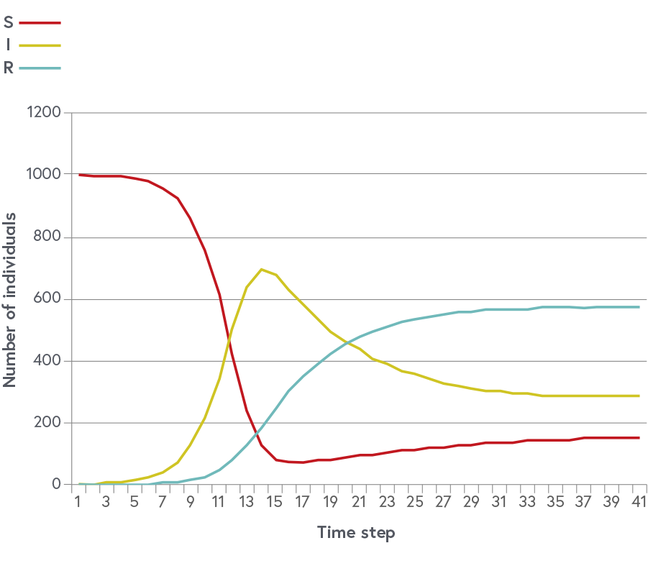## Want to keep learning?

This content is taken from the University of Basel's online course, One Health: Connecting Humans, Animals and the Environment. Join the course to learn more.
2.13

# Exercising with numbers: solution

Here you find the solution to the exercise of the previous step. If desired, you may download a completed spreadsheet.

The formulas should be:
$$S_{(T+1)}$$= B2 + ($F$2 * (B2+C2+D2)) - ($E$2 * B2 * C2) - ($F$2 * B2)
$$I_{(T+1)}$$= C2 + ($E$2 * B2 * C2) - ($F$2 * C2) - ($G$2 * C2)
$$R_{(T+1)}$$ = D2 + ($G$2 * C2) - ($F$2 * D2)

The graph should look like this:Time dependent changes in the numbers of susceptible, infectious and recovered individuals. © Jakob Zinsstag